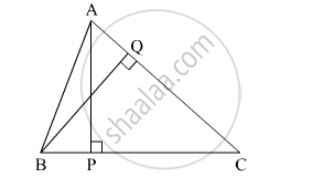# In ∆Abc, Ap ⊥ Bc, Bq ⊥ Ac B– P–C, A–Q – C Then Prove That, ∆Cpa ~ ∆Cqb. If Ap = 7, Bq = 8, Bc = 12 Then Find Ac. - Geometry

Sum

In ∆ABC, AP ⊥ BC, BQ ⊥ AC B– P–C, A–Q – C then prove that, ∆CPA ~ ∆CQB. If AP = 7, BQ = 8, BC = 12 then Find AC.#### Solution

n ∆CPA and ∆CQB,

∠CPA ≅ ∠CQB [Each angle is of measure 90°]

∠ACP ≅ ∠BCQ [Common angle]

∴ ∆CPA ~ ∆CQB [AA test of similarity]

therefore "AC"/"BC" = "AP"/"BQ"  [Corresponding sides of similar triangle]

therefore "AC"/12 = 7/8

therefore "AC" = "x" = (12 xx 7)/8

∴ AC = 10.5 units.

Concept: Similarity of Triangles
Is there an error in this question or solution?

#### APPEARS IN

Balbharati Mathematics 2 Geometry 10th Standard SSC Maharashtra State Board
Chapter 1 Similarity
Practice Set 1.3 | Q 4 | Page 21